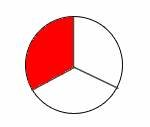Definition Of Denominator

The number below the bar in a fraction is called the Denominator.

We can also define denominator as the number of parts that a whole is divided into.

A fraction with zero (0) in the denominator is undefined.

Example of DenominatorThe given circle is divided into 3 parts. Out of the 3 parts, one part is shaded.
So, we write this model as in fraction form.
In this fraction 3 is the denominator whereas 1 is the numerator.

A. 39
B. 19
C. 40
D. 20